Pull Down & Release to Refresh

# Scatter Plots

'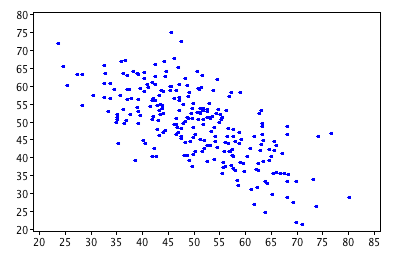A scatter plot is a diagram in the $xy$-plane that consists of a collection of plotted points. The points illustrate the relationship (if there is one) between two different sets of data, and are plotted as Cartesian coordinates.

In the example on the left, each point shows the marks of one student at Sam's school on two different tests.

Let's construct a scatter plot for an example.

### Example:Soup Sales

Sam's school canteen sells soup in terms 2 and 3. The canteen manager keeps track of the numbers of bowls of soup sold and the temperature each day. She hopes to be able to use the temperature to predict how much soup she should make any a given day. Here is the data from the last three weeks of school:

Soup Sales vs Temperature
Temperature (${}^\circ C$) Bowls of Soup Sold
8 28
10 25
11 24
8 26
12 22
15 18
8 27
17 15
16 20
12 21
21 9
16 18
17 15
18 12
20 8

Here's a scatter plot of the data: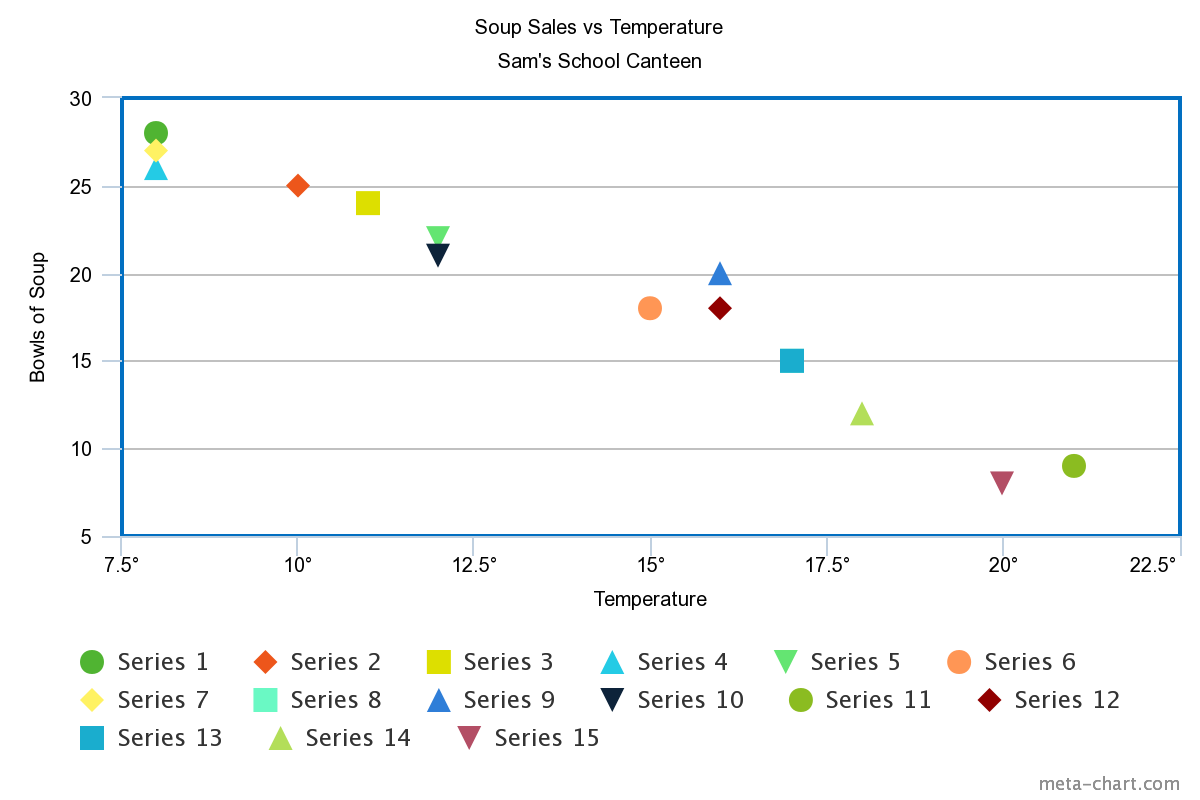The data appear to follow a straight line fairly closely, and the slope of the line is negative. So, it looks like the canteen manager should be able to use the temperature to predict how much soup to make. The relationship is not perfect, but it is easier to see that colder weather leads to more bowls of soup being sold.

## Line of Best Fit

We often draw a line of best fit (or trend line) to help us understand the relationship between the data sets plotted on our scatter plot. We choose the line that lies as close as possible to all of the points, and for which approximately the same numbers of points lie above and below the line.

Sometimes, it's enough to just estimate where the line should lie, but there are situations when we need to be more precise. We then use a technique called linear regression or least squares regression to find the line of best fit. We'll talk more about that in a more advanced article.

For our soup example, we don't need to be quite so precise. Here's a line of best fit drawn on the scatter plot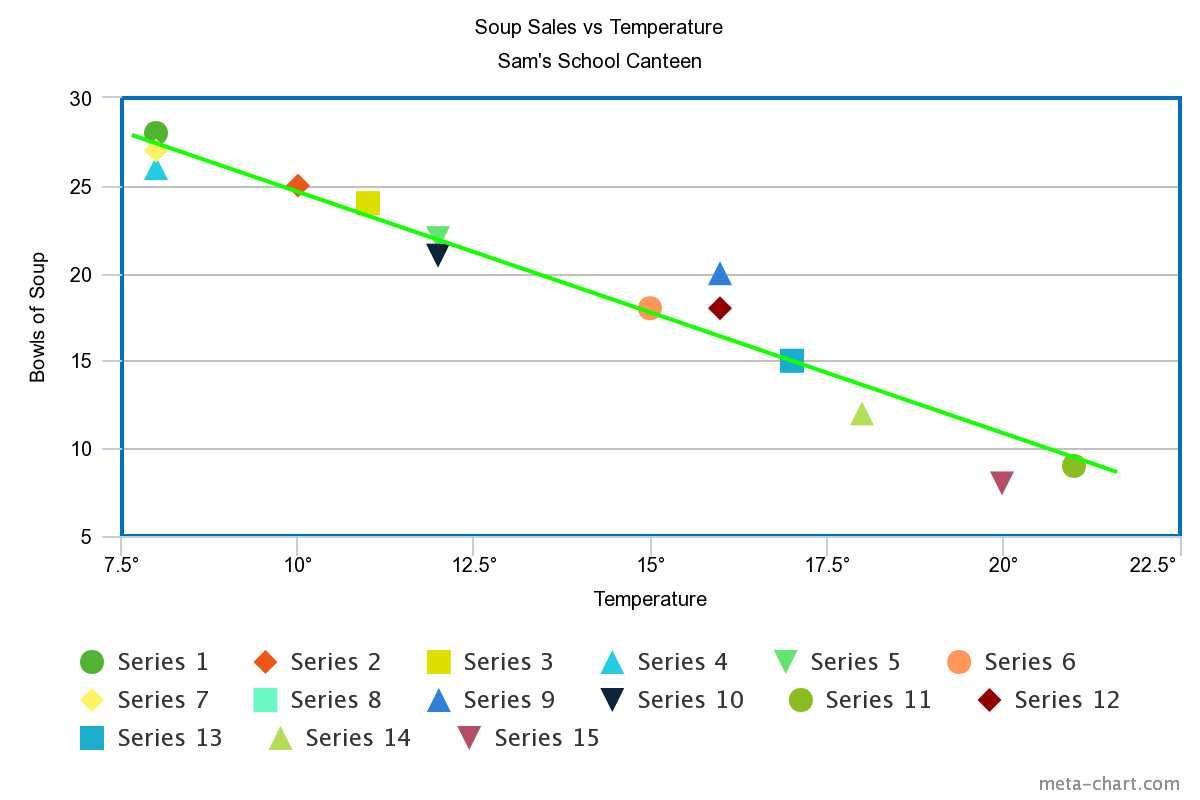Here's another example. Two data sets relating the stopping distances and speed of 1920s cars have been plotted on a scatter plot: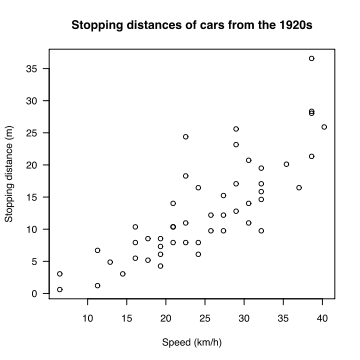I've had a go at drawing a line of best fit on the scatter plot. See if you can do better!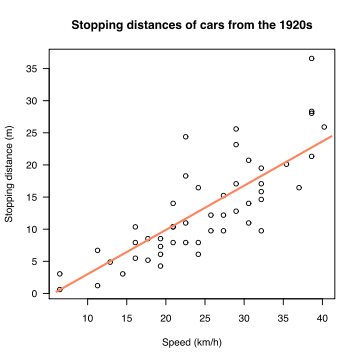## Interpolation and Extrapolation

In interpolation, we look for a missing value that lies in the range of our data set. For example, I have used linear interpolation (using a line to estimate the value) on the scatter plot below to estimate the number of bowls of soup sold when the temperature is $9 {}^\circ \text{C}\.)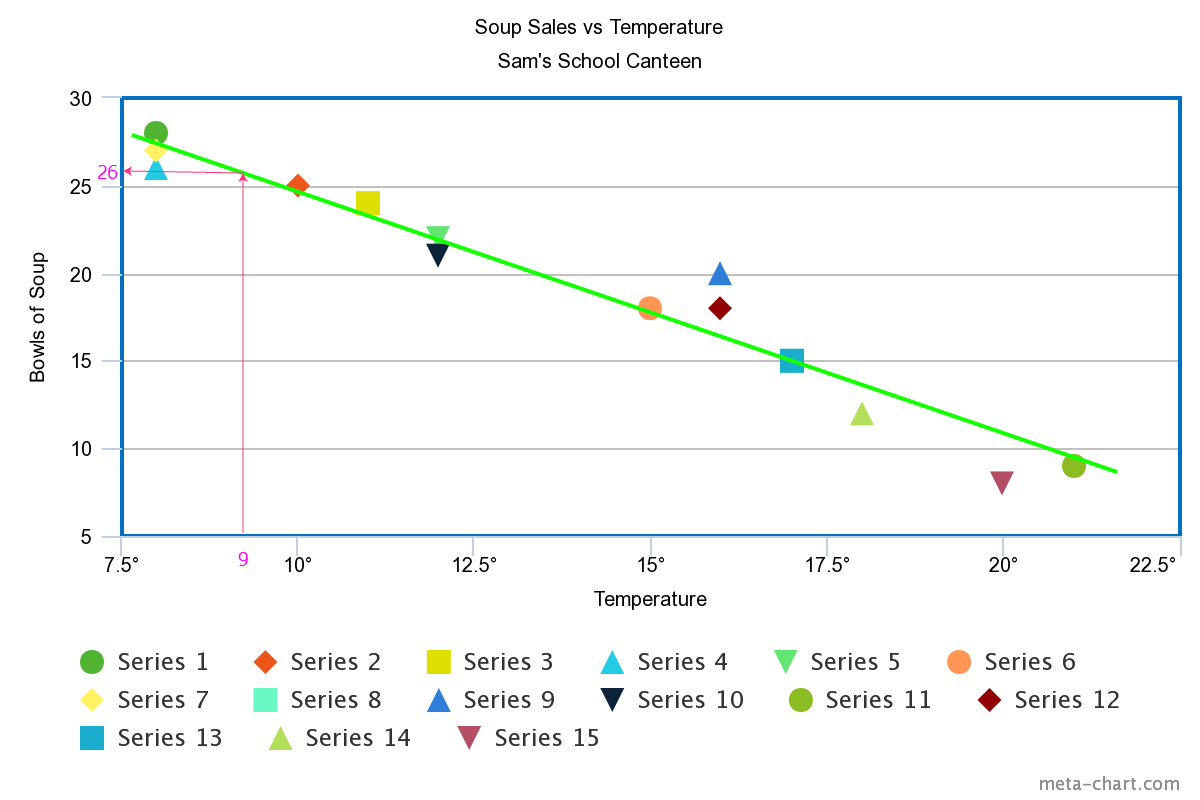In extrapolation, we look for a missing value that lies outside the range of our data set. We perform linear extrapolation by extending the line of best fit to include the data values we are looking for. On the scatter plot below, I've used linear extrapolation to estimate the number of bowls of soup sold when the temperature reaches \(22.5 {}^\circ \text{C}$.

Note: these techniques can only give an estimate of the missing values. Extrapolation, in particular, can give misleading results as we really can't be certain about what happens to our data values once we leave our data set.

### Using an Equation to Interpolate or Extrapolate

We can use the points on our scatter plot to come up with an approximate equation for the line of best fit. We can then use the equation of this line to extrapolate or interpolate.

Let's try it on our soup example. We only need two points to find the equation of a straight line. Choose two that are as close to the line of best fit as possible.

I've chosen the points $(15^\circ,18)$ and $(17^\circ, 15)$, corresponding to the orange circle and blue square on my scatter plot.

First, let's find the gradient (slope) of the line:

\begin{align*} \text{gradient} &= m = \dfrac{\text{change in }y }{\text{change in }x}\\ &= \dfrac{15 - 18}{17 - 15}\\ &= -\dfrac{3}{2} \end{align*}
Plug this gradient and the point $(15^\circ,18)$ into the point-gradient formula:
\begin{align*} y - y_1 &= m(x - x_1)\\ y - 18 &= - \dfrac{3}{2}(x - 15)\\ y - 18 &= - \dfrac{3}{2} \;x + 22.5\\ y &= -\dfrac{3}{2}\;x + 40.5 \end{align*}

#### Interpolating

We want to predict the number of bowls of soup that will be sold when the temperature is $9^\circ$, so we plug this $x$-value into the above equation to give

$y = -\dfrac{3}{2} (9) + 40.5 = 27$
The equation predicts $27$ bowls will be sold. This is quite close to the graphical prediction of $26$.

#### Extrapolating

If we want to predict the number of bowls of soup that will be sold when the temperature is $22.5^\circ$, then we need to extrapolate because this value is outside the range of our temperature data set. Plug $x = 22.5$ into the equation to give

$y = -\dfrac{3}{2} (22.5) + 40.5 = 6.75$
bowls, which is pretty close to the 7 we predicted with the graph.

You need to be very careful not to extrapolate too far. If you tried to use the equation to predict how many bowls of soup would be sold at a temperature of $40^\circ$, you'd get

$y = -\dfrac{3}{2} (40) + 40.5 = -19.5$,
which suggests that people are actually coming to the canteen and giving the manager bowls of soup. Of course, this is ridiculous: we've simply taken the extrapolation too far. Common sense suggests that they aren't going to be able to sell any soup if the temperature is $40 {}^\circ \text{C}$.

## Correlation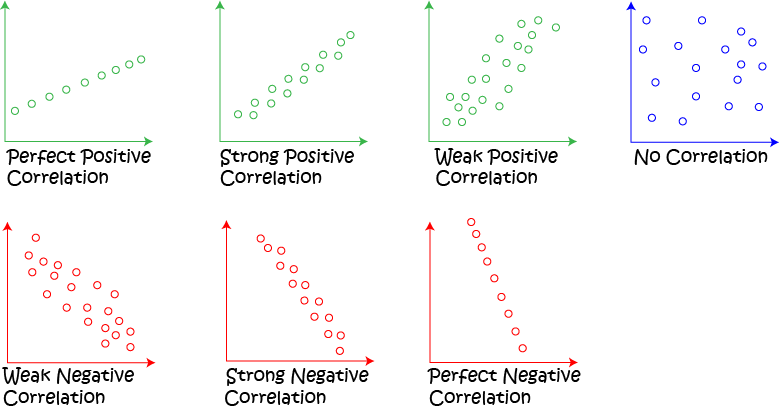Correlation gives us a measure of how strongly linked two sets of data are.

We say that the correlation is positive if both sets of data values increase together.

If one set of data values increases while the other decreases, then we say that the correlation is negative.

The values of linear correlation lie between $-1$ and $1$.

## Examples

There is a positive correlation between the stopping distances of 1920s cars and their speed:The stopping distance increases with the speed.

There is a negative correlation between soup sales and the temperature:The soup sales go down as the temperature goes up.

### Description

• Histograms
• Scatter plots
• Stem and leaf plots etc

these lessons are for students studying maths in Year 10 or highter

### Audience

Year 10 students or higher, however, suitable for Year 8+ students too.

### Learning Objectives

You must be logged in as Student to ask a Question.